Open in App
Not now

# How to Apply a function to multiple columns in Pandas?

• Last Updated : 16 Aug, 2022

Pandas.apply allow the users to pass a function and apply it on every single value of the Pandas series.

## Syntax of pandas.DataFrame.apply

Syntax : DataFrame.apply(parameters)

Parameters :

• func : Function to apply to each column or row.
• axis : Axis along which the function is applied
• raw : Determines if row or column is passed as a Series or ndarray object.
• result_type : ‘expand’, ‘reduce’, ‘broadcast’, None; default None
• args : Positional arguments to pass to func in addition to the array/series.
• **kwds : Additional keyword arguments to pass as keywords arguments to func.

Returns : Series or DataFrame

### Example 1: Pandas Apply Function to Single Column

In this example, we are passing only a single column and increment age with 2.

## Python3

 `# import the module` `import` `pandas as pd`   `# creating a DataFrame` `df ``=` `pd.DataFrame({``'String 1'``: [``'Tom'``, ``'Nick'``, ``'Krish'``, ``'Jack'``],` `                   ``'Age'``: [``32``, ``24``, ``33``, ``21``]})`   `# function for prepending 'Geek'` `def` `prepend_geek(age):` `    ``return` `age ``+` `2`   `# executing the function` `df[[``"Age"``]] ``=` `df[[``"Age"``]].``apply``(prepend_geek)`   `# displaying the DataFrame` `display(df)`

Output: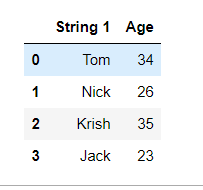### Example 2: Pandas Apply Function to multiple Columns

Here, we apply a function to two columns of Pandas Dataframe using Python concatenation.

## Python3

 `# import the module` `import` `pandas as pd`   `# creating a DataFrame` `df ``=` `pd.DataFrame({``'String 1'` `:[``'Tom'``, ``'Nick'``, ``'Krish'``, ``'Jack'``], ` `                   ``'String 2'` `:[``'Jane'``, ``'John'``, ``'Doe'``, ``'Mohan'``]})`   `# function for prepending 'Geek'` `def` `prepend_geek(name):` `    ``return` `'Geek '` `+` `name`   `# executing the function` `df[[``"String 1"``, ``"String 2"``]] ``=` `df[[``"String 1"``, ` `                                   ``"String 2"``]].``apply``(prepend_geek)`   `# displaying the DataFrame` `display(df)`

Output :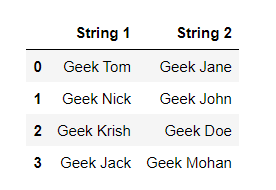### Example 3: Pandas Apply Function to All Columns

Here, we are multiplying all the columns by 2 by calling the multiply_by_2 function.

## Python3

 `# import the module` `import` `pandas as pd`   `# creating a DataFrame` `df ``=` `pd.DataFrame({``'Integers'` `:[``1``, ``2``, ``3``, ``4``, ``5``], ` `                   ``'Float'` `:[``1.1``, ``2.2``, ``3.3``, ``4.4` `,``5.5``],` `                  ``"Even_no"``:[``2``, ``4``, ``6``, ``8``, ``10``]})`   `# function for prepending 'Geek'` `def` `multiply_by_2(number):` `    ``return` `2` `*` `number`   `# executing the function` `df ``=` `df.``apply``(multiply_by_2)`   `# displaying the DataFrame` `display(df)`

Output :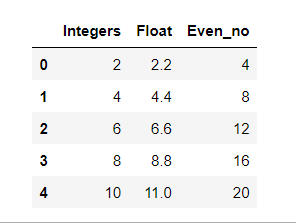### Example 4: Pandas Apply Function to single Columns using Numpy

In this example, we are applying panda’s apply function to only a single column i.e. Integers, and making it square using numpy.square.

## Python3

 `# import the module` `import` `pandas as pd` `import` `numpy as np`   `# creating a DataFrame` `df ``=` `pd.DataFrame({``'Integers'` `:[``1``, ``2``, ``3``, ``4``, ``5``], ` `                   ``'Float'` `:[``1.1``, ``2.2``, ``3.3``, ``4.4` `,``5.5``],` `                  ``"Even_no"``:[``2``, ``4``, ``6``, ``8``, ``10``]})`   `# executing the function` `df[``"Integers"``] ``=` `df[``"Integers"``].``apply``(np.square)`   `# displaying the DataFrame` `display(df)`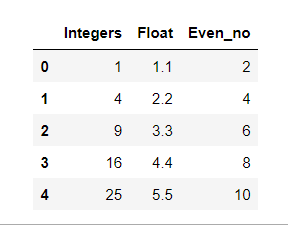### Example 5: Pandas Apply Function to All Columns using lambda

In this example, we are applying panda’s apply function to all the columns, and adding all the columns with 5 using Python lambda.

## Python3

 `# import the module` `import` `pandas as pd`   `# creating a DataFrame` `df ``=` `pd.DataFrame({``'Integers'` `:[``1``, ``2``, ``3``, ``4``, ``5``], ` `                   ``'Float'` `:[``1.1``, ``2.2``, ``3.3``, ``4.4` `,``5.5``],` `                  ``"Even_no"``:[``2``, ``4``, ``6``, ``8``, ``10``]})`   `# executing the function` `df ``=` `df.``apply``(``lambda` `x: x``+``5``)`   `# displaying the DataFrame` `display(df)`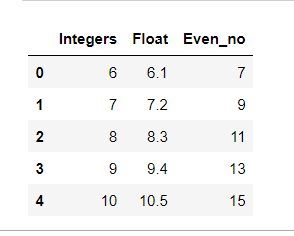My Personal Notes arrow_drop_up
Related Articles